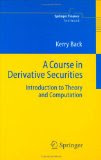• Hardcover: 372 pages
• Publisher: Springer; 1 edition (July 21, 2005)
• Language: English
• ISBN-10: 3540253734

This book aims at a middle ground between the introductory books on derivative securities and those that provide advanced mathematical treatments. It is written for mathematically capable students who have not necessarily had prior exposure to probability theory, stochastic calculus, or computer programming. It provides derivations of pricing and hedging formulas (using the probabilistic change of numeraire technique) for standard options, exchange options, options on forwards and futures, quanto options, exotic options, caps, floors and swaptions, as well as VBA code implementing the formulas. It also contains an introduction to Monte Carlo, binomial models, and finite-difference methods.

http://w13.easy-share.com/2577121.html